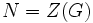# No common composition factor with quotient group not implies complemented

This article gives the statement and possibly, proof, of a non-implication relation between two subgroup properties. That is, it states that every subgroup satisfying the first subgroup property (i.e., normal subgroup having no common composition factor with its quotient group) need not satisfy the second subgroup property (i.e., complemented normal subgroup)
View a complete list of subgroup property non-implications | View a complete list of subgroup property implications
Get more facts about normal subgroup having no common composition factor with its quotient group|Get more facts about complemented normal subgroup
EXPLORE EXAMPLES YOURSELF: View examples of subgroups satisfying property normal subgroup having no common composition factor with its quotient group but not complemented normal subgroup|View examples of subgroups satisfying property normal subgroup having no common composition factor with its quotient group and complemented normal subgroup

## Statement

It is possible to have a finite group$G$ having a normal subgroup$N$ such that$N$ and$G/N$ have no common composition factors, but$N$ is not a permutably complemented subgroup of$G$.

## Proof

Further information: special linear group:SL(2,5)

Let$G = SL(2,5)$, the special linear group of$2 \times 2$ matrices over the field of five elements. Let$N = Z(G)$. Then,$N$ is a subgroup of order two and$G/N$ is isomorphic to the alternating group of degree five, which is simple. Thus,$N$ and$G/N$ have no common composition factors. However,$N$ has no complement in$G$.